## Saturday, 10 September 2011

### Determinants for M2 II: alternative techniques

Determinants

The cyclic determinants are most properly in these forms: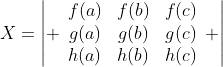, or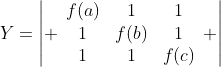, or ANY determinants that can reach these forms via elemental operations. Their symmetricities can be proven by direct expansion so I will skip this.

Here comes the most important point : Det(X) is NOT symmetrical, it's asymmetrical because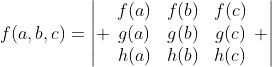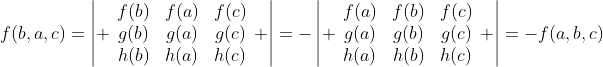But it's cyclic since putting f(a,b,c) to f(b,c,a) involve two times of interchanging rows, we will skip this part.

At the same time, Y is symmetrical because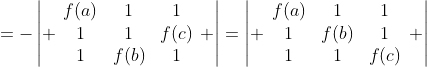And now we will discuss an alternative technique in factorizing determinants.
By factor theorem, if f(a)=0, then (x-a) is a factor of f. Similar we state determinants as a polynomial function, for 2 variables a,b in the determinants which a=b (or other condition), then (a-b) is a factor of it.
Example 1: Factorize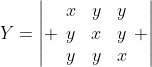.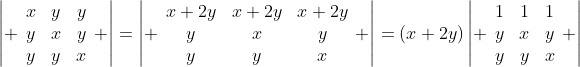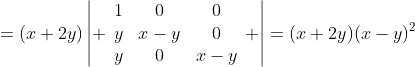Some students think that it's difficult to reach the idea to add up the rows, so you can factorize some easier component first:
Since when x=y, the three rows (and at least two of it) are identical. Therefore (x-y) must be a factor of the determinant.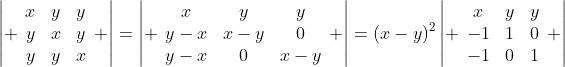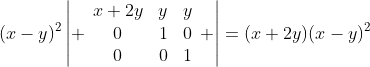We used different approach, but the answer's still unique.
In this example I've demonstrated in a symmetrical-like (even some of its factor is not symmetrical) determinants how factorization can be easier.

Example 2: Factorize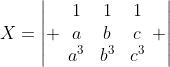.
This is a classic example. I have proven that this is asymmetrical, i.e., X=0 if a=b or b=c or c=a. Therefore (a-b)(b-c)(c-a) is a factor of x. Equating the degree,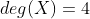but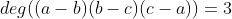, and the only three variable first degree cyclic function is (a+b+c), we have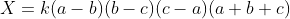. Comparing the coefficient of a certain term, say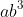, is 1 by expanding det(X) therefore k = 1. So X=(a-b)(b-c)(c-a)(a+b+c).

For determinants in this form it can be factorized into one product nicely. By measuring its degree and asymmetrical properties, factorizing can be much easier.

Exercises:
1) Solve the following problems about determinants:
a) Show without expanding that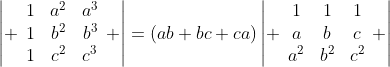(Hint: consider the transpose of determinants)
b) Show that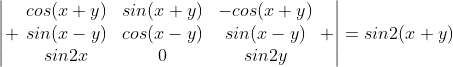c) Factorize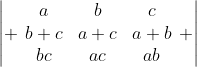.
d) Show without expanding that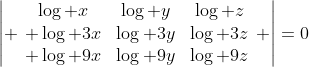e) Show without expanding that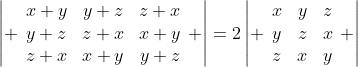f) Factorize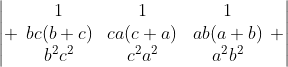.

Reference:
1) My project(2010): Basic Algebraic Skills, p.46-49 "Multivariate function". You're also welcomed to finish the exercise there.
2) Margaret M. Gow(1960), A Course in Pure Mathematics p.33, problems may or may not modified from here.

These problems about determinants are actually quite hard, so if you can do them without difficulties, probably you hv been mastered this topic!